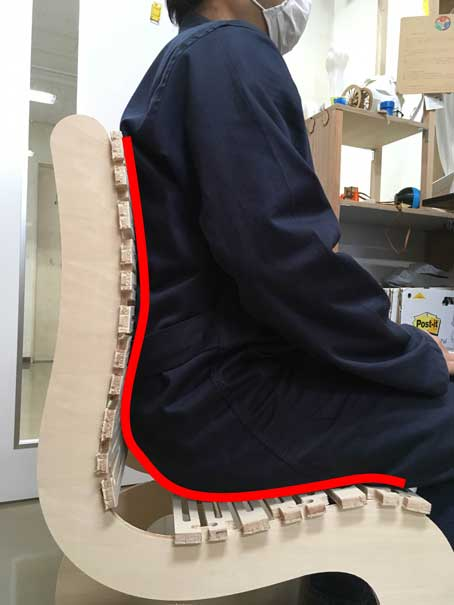# Week8 : Computer-Controlled Machining

## Group Work

We learn about shop bot charactaristic in group work. Our group work is here.

## Make Something Big

### ■I have not find best chair for me

I have low back pain and stiff shoulders. I think that these problems can be solved by proper posture.For that reason, I have spent a lot of money on chairs in my life, expensive chair, ergornomics design chair..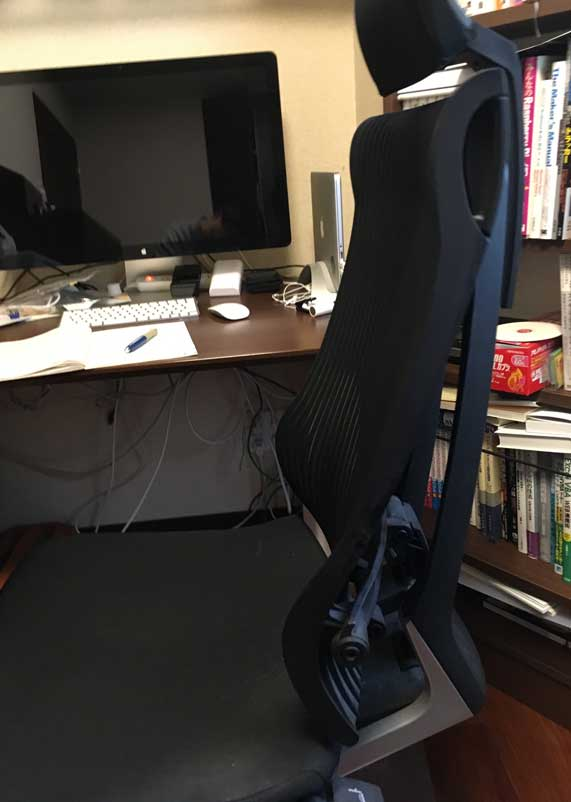Most of the chairs have bulging in the waist and the upper part has a slope.These design is good for leaning and resting. But, if I use the chair for desk work,these chair doesn't support my body.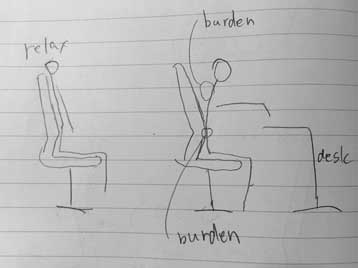In my opinion bulging should be placed more upper and giving a slope to the sitting part.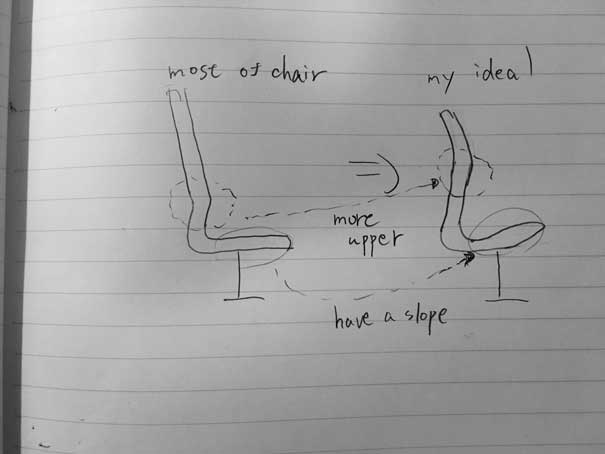【Effect】
• Upper bulding : Posture is surely better than that, and no burden is placed on the neck and back.(You can't bend the back because of this bulding.)
• Sitting part slope : Stabilize the pelvis by giving a slope to the sitting part.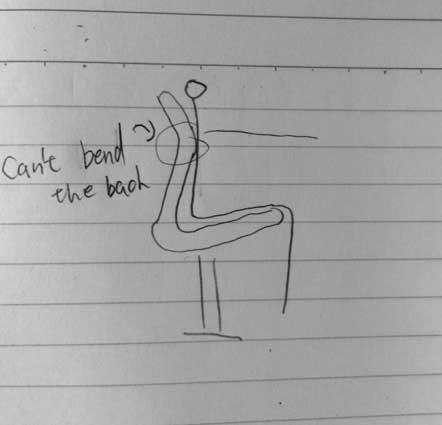【My Size】
So, I decided to make the my best chair that is good for low back pain and stiff shoulders with living hinge. To fit my body, bulging part should be placed 290mm from the seat surface and be 25-30mm hight.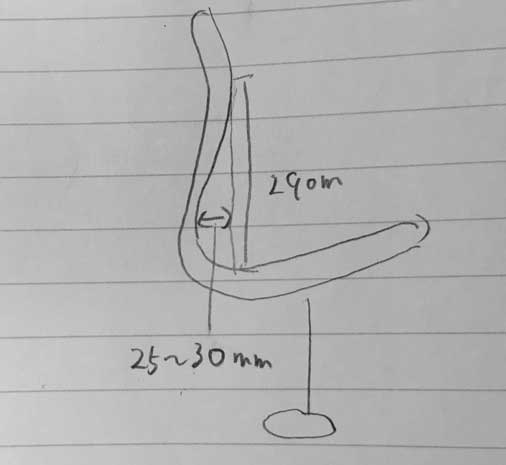### ■Parametric Design

I made living hinge generator because I felt it would be very troublesome to change living hinge design.After studying Nicolas' review of Week 4, I studied javascript, so I decided to make it with javascript.

### ■Living Hinge Generator with Java script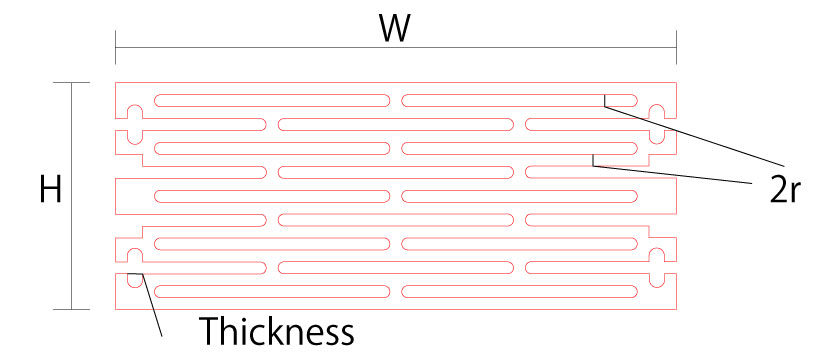r:mm
W:mm
H:mm
Thikness:mm
Offset:mm
Thikness Offset:mm

#### ■How to make Living Hinge Generator with Java script

Java script can be write with text editor. I use Atom. I wrote the following program with java script and upload it to the gitlab.
``````                                    function calsvg(){
var y0,xo1,yo1,xo2,xo2l,yo2,xo3,xo3l,yo3,xo4,yo4;
var x,y,x0;
var h,r,L,l,mg,mro,gap;
var th,sm,sd,tho;
var cw,ch,ww,wh;
var sti1,sti2,st1,st2,st3;
var result_w,result_h;
var temp;
var ed1,ed2;
var lp,s;
var convex,between_convex;
var offset,os_offset;
var thoffset;
var ifover;
ww=Number(par_form.form_w.value);
wh=Number(par_form.form_h.value);
th=Number(par_form.form_t.value);
offset=Number(par_form.form_off.value);
thoffset=Number(par_form.form_thoff.value);

os_offset=offset/2;
tho=th+thoffset;//offset込みの厚さ

r=Number(par_form.form_r.value);//6;//th/2;
sm=2*r;//ヘリからスリットまでのマージン

cw=ww+20;
ch=wh+5*r;

st1 = "<svg xmlns=\"http://www.w3.org/2000/svg\" version=\"1.1\" width=\""+cw+"mm\" height=\""+ch+"mm\" viewBox=\"0 0 "+cw+" "+ch+"\">";
ed1 = "</svg>";

//sti1 = "<path stroke=\"black\" stroke-width=\"0.5\" fill=\"none\" d=\"M0,0 H"+cw+" V"+ch+" H 0 V0 \"/>";
//sti2 = "<path stroke=\"black\" stroke-width=\"0.5\" fill=\"none\" d=\"M"+cw/2+",0 V"+ch+" \"/>";//centerline

L=(ww-2*r-2*tho-2*sm-4*r)/2;//一つの穴の長さ(スリットとの間に4r開けたい＆二つ並ぶ時２r開けたい)
l=L-2*r;//円弧を抜いた長さ
mg=ww/2-L;//ww/2*(1-mro);//マージン
gap=2*r//mg/4;

s=Math.floor((wh+10*r)/(24*r));
lp=Number((s-1)*3+2);

result_h=24*r*s-10*r;
ifover=24*r*(s+1)-10*r;

sd=r;//depth of slit

convex=2*r+2*sd;//
between_convex=22*r-2*sd;//

//set(0,0)------------------------
x0=cw/2-ww/2;
y0=2*r;
//--------------------------------
//--------------------------------------
//base shape
xo1=r;
yo1=0;
xo2=xo1+l;
xo2l=xo1+l+r+tho;
yo2=yo1;
xo3=xo2;
xo3l=xo2l;
yo3=yo1+2*r;
xo4=xo1;
yo4=yo3;
//--------------------------------------
for(var i=1;i<=lp;i++){
if(i==1){
y1=0;
}else{
//中央配置
x1=cw/2-L/2;
y1=y2+4*r
temp = "<path stroke=\"red\" stroke-width=\"0.5\" fill=\"none\" d=\"M"+xo1+","+yo1+" H"+xo2+" A "+r+" "+r+" 0 0 1 "+xo3+" "+yo3+" H"+xo4+" A "+r+" "+r+" 0 0 1 "+xo1+" "+yo1+"\" transform=\"translate("+x1+","+y1+")\"/>";
st2=st2 + "\n" + temp;
}
if((i % 3)!=0){
x2=cw/2-L-gap/2;
y2=y1+4*r;
temp = "<path stroke=\"red\" stroke-width=\"0.5\" fill=\"none\" d=\"M"+xo1+","+yo1+" H"+xo2+" A "+r+" "+r+" 0 0 1 "+xo3+" "+yo3+" H"+xo4+" A "+r+" "+r+" 0 0 1 "+xo1+" "+yo1+"\" transform=\"translate("+x2+","+y2+")\"/>";
st2=st2 + "\n" + temp;

x3=cw/2+gap/2;
y3=y1+4*r;
temp = "<path stroke=\"red\" stroke-width=\"0.5\" fill=\"none\" d=\"M"+xo1+","+yo1+" H"+xo2+" A "+r+" "+r+" 0 0 1 "+xo3+" "+yo3+" H"+xo4+" A "+r+" "+r+" 0 0 1 "+xo1+" "+yo1+"\" transform=\"translate("+x3+","+y3+")\"/>";
st2=st2 + "\n" + temp;

}else{
/*
x2=x0+sm;
y2=y1+4*r;
temp = "<path stroke=\"red\" stroke-width=\"0.5\" fill=\"none\" d=\"M"+xo1+","+yo1+" H"+xo2l+" A "+r+" "+r+" 0 0 1 "+xo3l+" "+yo3+" H"+xo4+" A "+r+" "+r+" 0 0 1 "+xo1+" "+yo1+"\" transform=\"translate("+x2+","+y2+")\"/>";
st2=st2 + "\n" + temp;

x3=cw/2+gap/2;
y3=y1+4*r;
temp = "<path stroke=\"red\" stroke-width=\"0.5\" fill=\"none\" d=\"M"+xo1+","+yo1+" H"+xo2l+" A "+r+" "+r+" 0 0 1 "+xo3l+" "+yo3+" H"+xo4+" A "+r+" "+r+" 0 0 1 "+xo1+" "+yo1+"\" transform=\"translate("+x3+","+y3+")\"/>";
st2=st2 + "\n" + temp;
*/
x2=cw/2-L-gap/2;
y2=y1+4*r;
temp = "<path stroke=\"red\" stroke-width=\"0.5\" fill=\"none\" d=\"M"+xo1+","+yo1+" H"+xo2+" A "+r+" "+r+" 0 0 1 "+xo3+" "+yo3+" H"+xo4+" A "+r+" "+r+" 0 0 1 "+xo1+" "+yo1+"\" transform=\"translate("+x2+","+y2+")\"/>";
st2=st2 + "\n" + temp;

x3=cw/2+gap/2;
y3=y1+4*r;
temp = "<path stroke=\"red\" stroke-width=\"0.5\" fill=\"none\" d=\"M"+xo1+","+yo1+" H"+xo2+" A "+r+" "+r+" 0 0 1 "+xo3+" "+yo3+" H"+xo4+" A "+r+" "+r+" 0 0 1 "+xo1+" "+yo1+"\" transform=\"translate("+x3+","+y3+")\"/>";
st2=st2 + "\n" + temp;
}
}
//--------------------------------------

temp="";

x=x0;
y=y0;
st3 = "<path stroke=\"red\" stroke-width=\"0.5\" fill=\"none\" d=\"M"+x+","+y ;
ed2="\"/>";
y=y+6*r;
temp=temp+" V"+y;//↓

for(var i=1;i<lp;i++){

if ((i%3)==1){
//slit-------------
x=x0+sm;
temp=temp+" H"+x;//→
y=y-sd-os_offset;
temp=temp+" V"+y;//↑
x=x+tho;
temp=temp+" A "+r+" "+r+" 0 0 1 "+x+" "+y//∩
y=y+sd+os_offset;
temp=temp+" V"+y;//↓
//-------------
x=cw/2-L/2-3*r;
temp=temp+" H"+x;//→→→→→→→→
y=y+2*r;
temp=temp+" A "+r+" "+r+" 0 0 1 "+x+" "+y//コ
//temp=temp+" V"+y;

//slit-------------
x=x0+sm+tho;
temp=temp+" H"+x;//←
y=y+sd+os_offset;
temp=temp+" V"+y;//↓
x=x-tho;
temp=temp+" A "+r+" "+r+" 0 0 1 "+x+" "+y//U
y=y-sd-os_offset;
temp=temp+" V"+y;//↑
//-------------
x=x0;
temp=temp+" H"+x;//←
if (i!=(lp-1)){
y=y+4*r;
}else{
y=y+6*r;
}
temp=temp+" V"+y;//↓
}else{

if ((i%3)==2){
x=x0+sm+tho;
temp=temp+" H"+x;//→→→→
y=y+2*r;
temp=temp+" V"+y;//↓
x=cw/2-L/2-3*r;
temp=temp+" H"+x;//→→→→→→→→
y=y+2*r;
temp=temp+" A "+r+" "+r+" 0 0 1 "+x+" "+y//コ
x=x0;
temp=temp+" H"+x;//←←←←←←

y=y+6*r;
temp=temp+" V"+y; //↓
}

if ((i%3)==0){
x=cw/2-L/2-3*r;
temp=temp+" H"+x;//→→→→→→→→
y=y+2*r;
temp=temp+" A "+r+" "+r+" 0 0 1 "+x+" "+y//コ
x=x0+sm+tho;
temp=temp+" H"+x;//←←←←←←←←
y=y+2*r;
temp=temp+" V"+y; //↓
x=x0;
temp=temp+" H"+x;//←←←
y=y+4*r;
temp=temp+" V"+y;    //↓
}

}
}
x=cw/2+ww/2;;
temp=temp+" H"+x;
for(var i=1;i<lp;i++){
if ((i%3)==1){
if(i!=1){
y=y-4*r;
}else{
y=y-6*r;
}
temp=temp+" V"+y;//↑
//slit-------------
x=x-sm;
temp=temp+" H"+x;//←
y=y+sd+os_offset;
temp=temp+" V"+y;//↓
x=x-tho;
temp=temp+" A "+r+" "+r+" 0 0 1 "+x+" "+y//U
y=y-sd-os_offset;
temp=temp+" V"+y;//↑
//-------------
x=cw/2+L/2+3*r;
temp=temp+" H"+x;//←←←←←←←←
y=y-2*r;
temp=temp+" A "+r+" "+r+" 0 0 1 "+x+" "+y//<
//temp=temp+" V"+y;
//slit-------------
x=cw/2+ww/2-sm-tho;
temp=temp+" H"+x;//→→→→→→→→
y=y-sd-os_offset;
temp=temp+" V"+y;//↑
x=x+tho;
temp=temp+" A "+r+" "+r+" 0 0 1 "+x+" "+y//∩
y=y+sd+os_offset;
temp=temp+" V"+y;//↓
x=cw/2+ww/2;
temp=temp+" H"+x;//→

}else{
if (i%3==2){
y=y-4*r;
}else{
y=y-6*r;
}
temp=temp+" V"+y;//↑

if ((i%3)==2){
x=cw/2+ww/2-sm-tho;
temp=temp+" H"+x;
y=y-2*r;
temp=temp+" V"+y;
}
x=cw/2+L/2+3*r;
temp=temp+" H"+x;
y=y-2*r;
temp=temp+" A "+r+" "+r+" 0 0 1 "+x+" "+y

if((i%3)==0){
x=cw/2+ww/2-sm-tho;
temp=temp+" H"+x;
y=y-2*r;
temp=temp+" V"+y;
}
x=cw/2+ww/2;
temp=temp+" H"+x;

}
}
y=y0;
temp=temp+" V"+y;
x=x0;
temp=temp+" H"+x;

st3=st3+temp+ed2;

//-------------------------------------------------------
var alstr;
alstr="Set your convex-width:"+convex+"mm.\nBetween convex surfaces"+between_convex+"mm.\nYour request hight was "+wh+"mm. Result hight is "+result_h+"mm";
if (result_h<wh){
alstr=alstr+"\nIf you need at least" +wh+"mm.The hight should be set" +ifover+"mm.";
}
var svgtxt = st1;
svgtxt = svgtxt + "\n" + sti1;
svgtxt = svgtxt + "\n" + sti2;
svgtxt = svgtxt + "\n" + st2;
svgtxt = svgtxt + "\n" + st3;
svgtxt = svgtxt + "\n" + ed1;

var blob = new Blob([ svgtxt ], { "type" : "text/plain" });

if (window.navigator.msSaveBlob) {
window.navigator.msSaveBlob(blob, "LivingHinge.svg");

// msSaveOrOpenBlobの場合はファイルを保存せずに開ける
window.navigator.msSaveOrOpenBlob(blob, "LivingHinge.svg");
} else {
}

}``````

### ■Other Parts Design & Design Motif

The parts other than Living hinge were designed with Fusion 360. The design was exported to DXF format beforer using Shop Bot.
My final project is related with ocean, so I designed parts with waves as a motif so that poeple can associate with the ocean.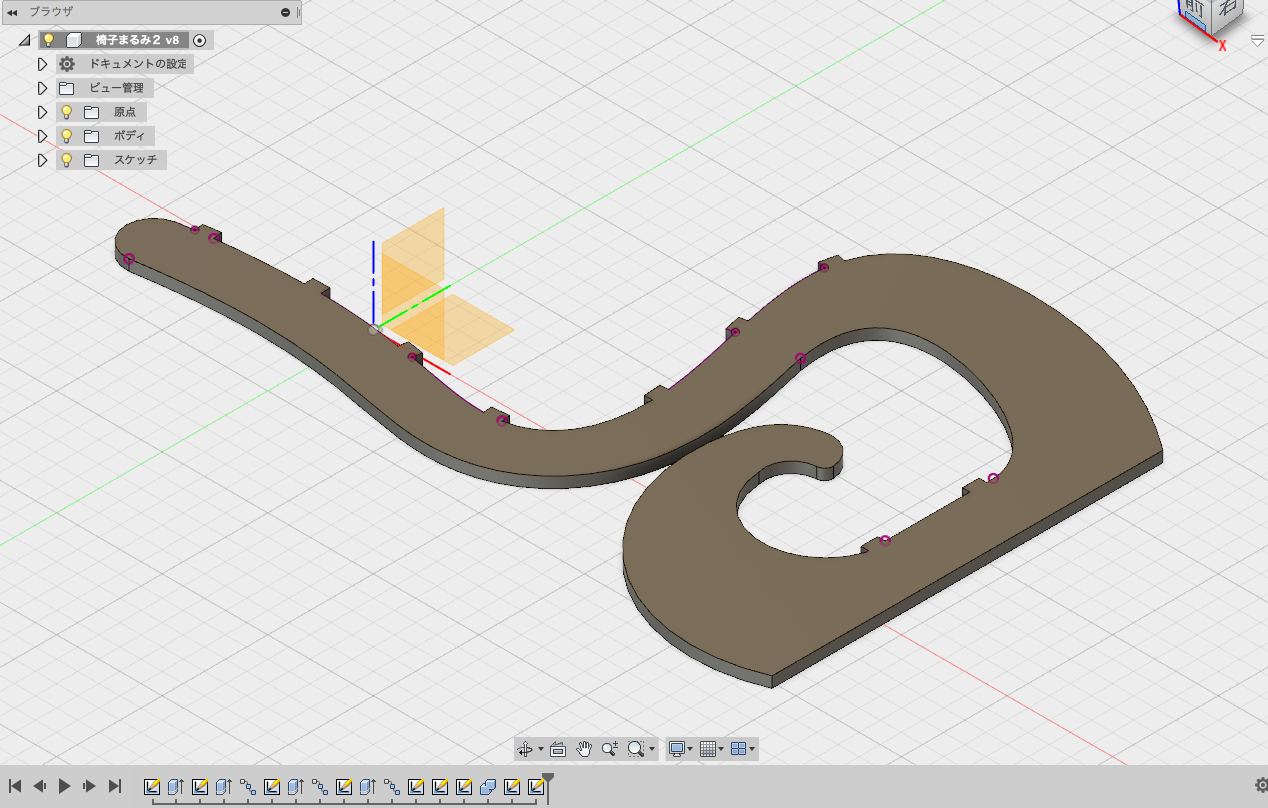### ■Living Hinge Test

I should had to know how degree the living hinge could be bendl, so I tested that. By this test it could be bend about 45°. I had intended to make it with one living hinge board at first, but it turned out that it was impossible. So, I decided to devide living hinge parts as back part and sitting part.

### ■Shop Bot

As there isn't Big CNC router at Fablab Kamakura, we went to VUILD to use Shop Bot. So, we had a constraint that we could use Shop Bot for only one day.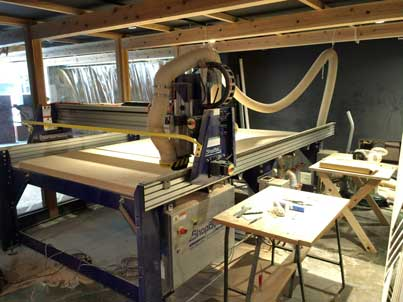【Shop Bot Settings】

【Vcurve Pro -> Shopbot】
We use Vcurve Pro for CAM software. 【Tool】
We use o.25 inch diameter flat endmill. Feed rate was 2.5 inch/sec. spindle speed was 14000 rev / min.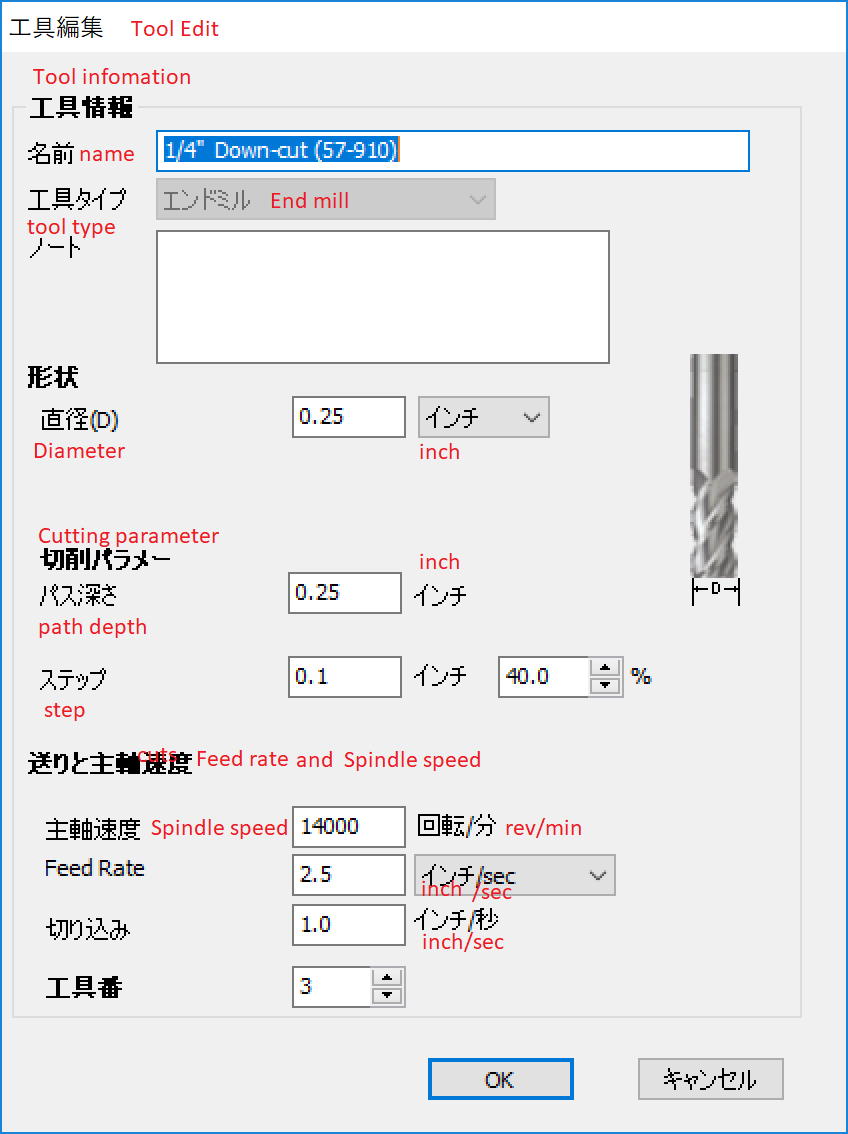【Fix the material】
The material(plywood big sheet) was fixed with screws.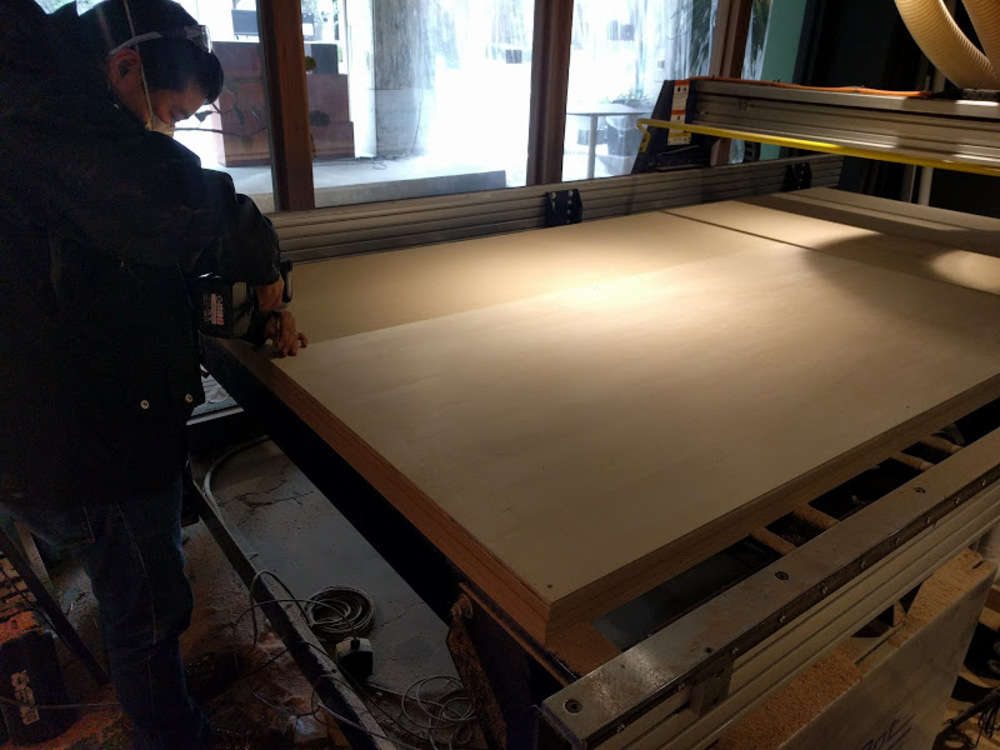【Test Pars】
We made some test parts to recongnize the actural size. By this test, I decided offset to 0.3mm.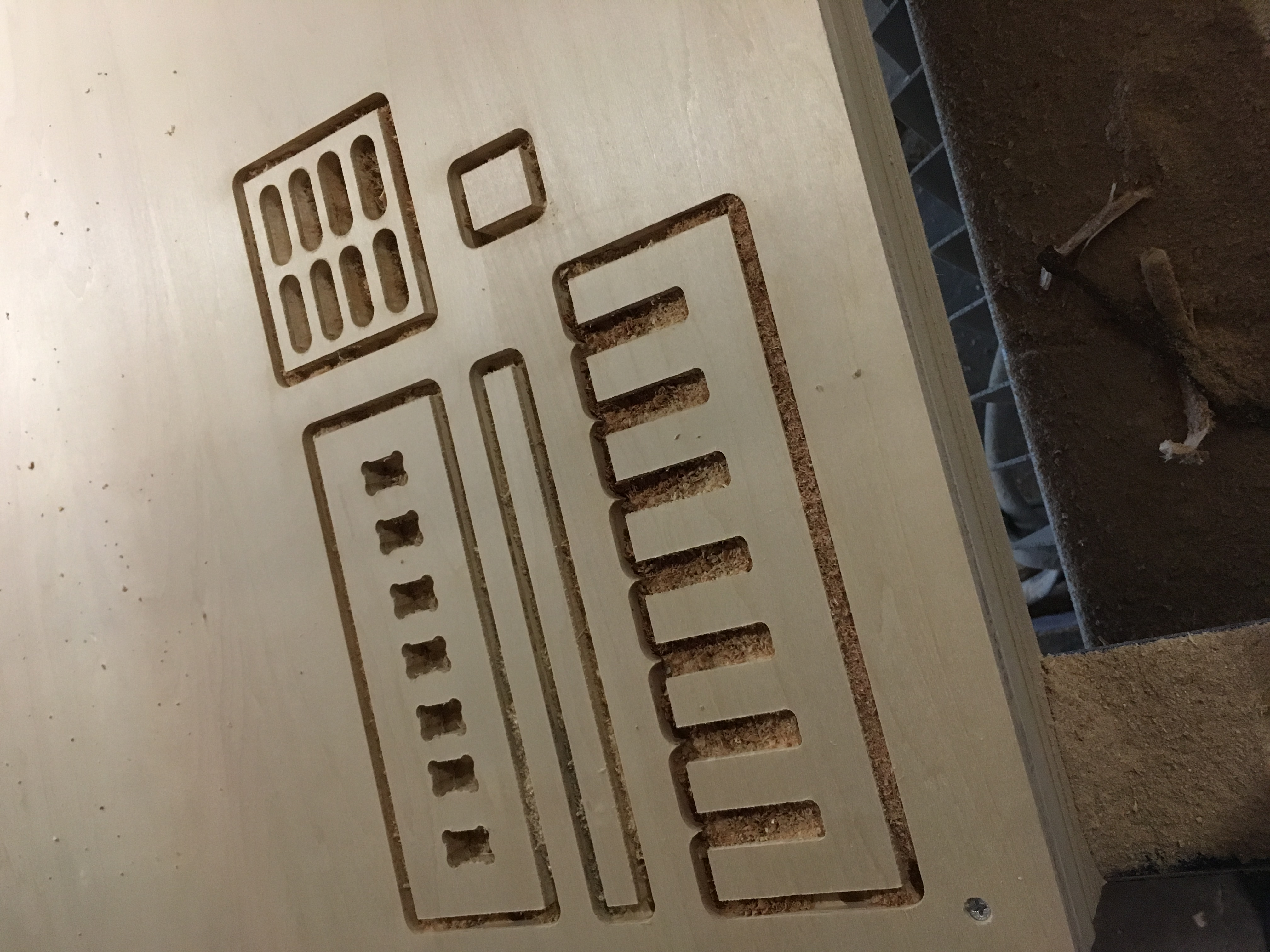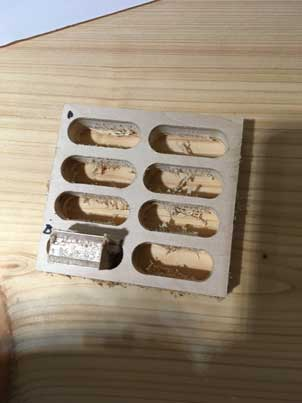【Cut】
Material : Plywood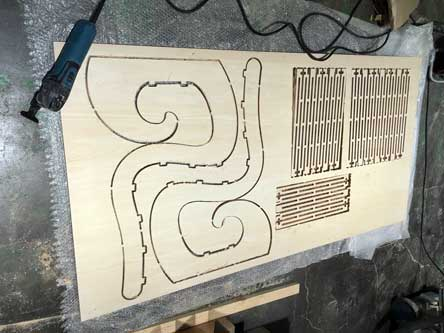### ■Problem in Vcurve

My desing which is made with Fusion 360 was couldn't made tool path because of its vector was opened. This problem was solved by unite vector function(edit -> unite vector) in Vcurve.
My desing which is made with Fusion 360 was couldn't made tool path because of its vector was opened. This problem was solved by unite vector function(edit -> unite vector) in Vcurve.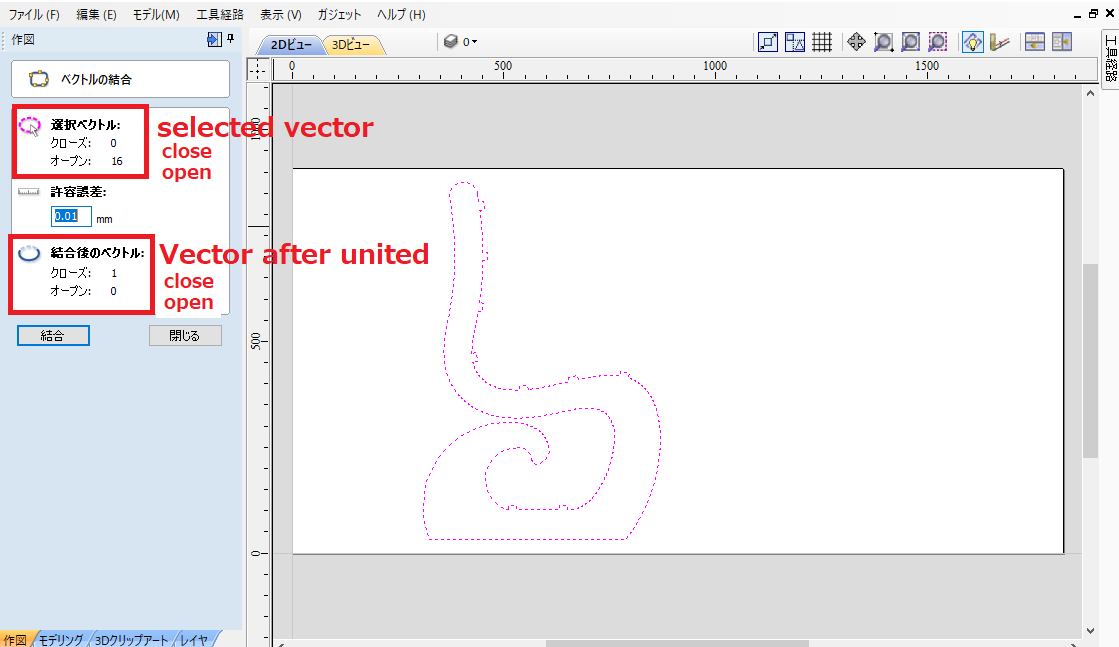### ■Extra:Laser Cutter could be used for cutting the wood?

I wondered if the laser cutter could be used for cutting wood. I tried to cut with laser cutter. It is difficult to cut at once. So, I made the drawing line-symmetrical and cut it again from the back, it was beautifully cut. But, cutting surfaces are scorched considerably.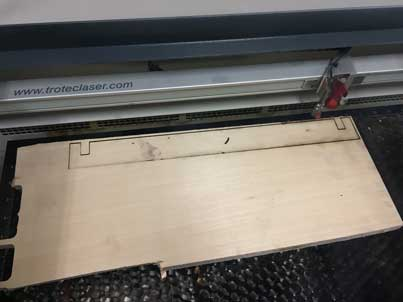### ■Finish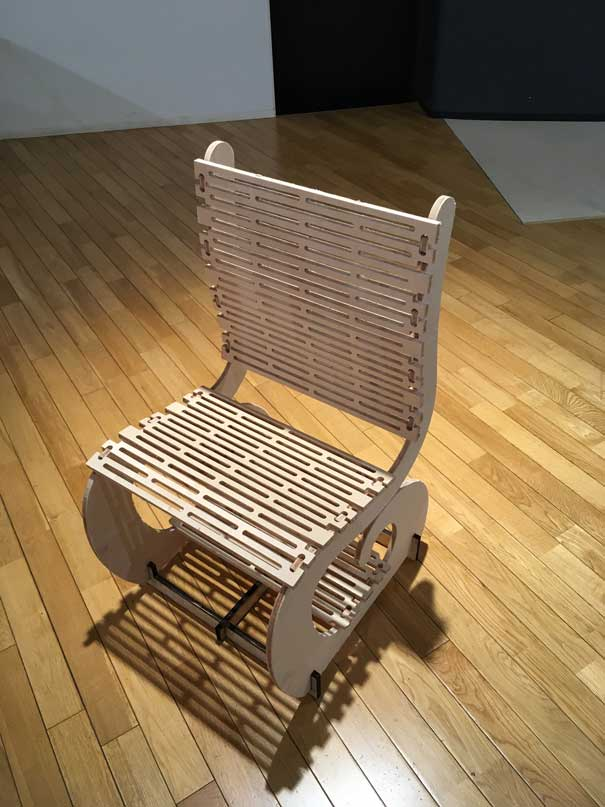### ■Finally I met the best chair for me!

This chair is perfectly fitted to my body, so I can sit in the right posture, stiff shoulders and back pain will disappear!